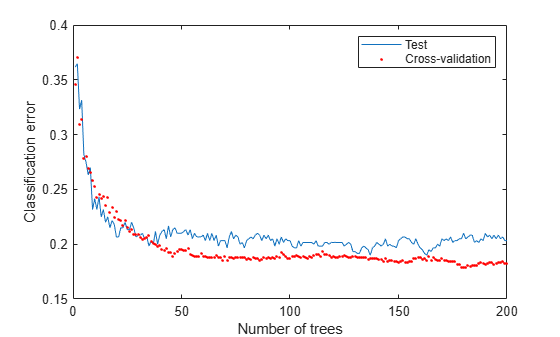# Test Ensemble Quality

You cannot evaluate the predictive quality of an ensemble based on its performance on training data. Ensembles tend to "overtrain," meaning they produce overly optimistic estimates of their predictive power. This means the result of `resubLoss` for classification (`resubLoss` for regression) usually indicates lower error than you get on new data.

To obtain a better idea of the quality of an ensemble, use one of these methods:

• Evaluate the ensemble on an independent test set (useful when you have a lot of training data).

• Evaluate the ensemble by cross validation (useful when you don't have a lot of training data).

• Evaluate the ensemble on out-of-bag data (useful when you create a bagged ensemble with `fitcensemble` or `fitrensemble`).

This example uses a bagged ensemble so it can use all three methods of evaluating ensemble quality.

Generate an artificial dataset with 20 predictors. Each entry is a random number from 0 to 1. The initial classification is $Y=1$ if ${X}_{1}+{X}_{2}+{X}_{3}+{X}_{4}+{X}_{5}>2.5$ and $Y=0$ otherwise.

```rng(1,'twister') % For reproducibility X = rand(2000,20); Y = sum(X(:,1:5),2) > 2.5;```

In addition, to add noise to the results, randomly switch 10% of the classifications.

```idx = randsample(2000,200); Y(idx) = ~Y(idx);```

### Independent Test Set

Create independent training and test sets of data. Use 70% of the data for a training set by calling `cvpartition` using the `holdout` option.

```cvpart = cvpartition(Y,'holdout',0.3); Xtrain = X(training(cvpart),:); Ytrain = Y(training(cvpart),:); Xtest = X(test(cvpart),:); Ytest = Y(test(cvpart),:);```

Create a bagged classification ensemble of 200 trees from the training data.

```t = templateTree('Reproducible',true); % For reproducibility of random predictor selections bag = fitcensemble(Xtrain,Ytrain,'Method','Bag','NumLearningCycles',200,'Learners',t)```
```bag = ClassificationBaggedEnsemble ResponseName: 'Y' CategoricalPredictors: [] ClassNames: [0 1] ScoreTransform: 'none' NumObservations: 1400 NumTrained: 200 Method: 'Bag' LearnerNames: {'Tree'} ReasonForTermination: 'Terminated normally after completing the requested number of training cycles.' FitInfo: [] FitInfoDescription: 'None' FResample: 1 Replace: 1 UseObsForLearner: [1400x200 logical] Properties, Methods ```

Plot the loss (misclassification) of the test data as a function of the number of trained trees in the ensemble.

```figure plot(loss(bag,Xtest,Ytest,'mode','cumulative')) xlabel('Number of trees') ylabel('Test classification error')```### Cross Validation

Generate a five-fold cross-validated bagged ensemble.

`cv = fitcensemble(X,Y,'Method','Bag','NumLearningCycles',200,'Kfold',5,'Learners',t)`
```cv = ClassificationPartitionedEnsemble CrossValidatedModel: 'Bag' PredictorNames: {1x20 cell} ResponseName: 'Y' NumObservations: 2000 KFold: 5 Partition: [1x1 cvpartition] NumTrainedPerFold: [200 200 200 200 200] ClassNames: [0 1] ScoreTransform: 'none' Properties, Methods ```

Examine the cross-validation loss as a function of the number of trees in the ensemble.

```figure plot(loss(bag,Xtest,Ytest,'mode','cumulative')) hold on plot(kfoldLoss(cv,'mode','cumulative'),'r.') hold off xlabel('Number of trees') ylabel('Classification error') legend('Test','Cross-validation','Location','NE')```Cross validating gives comparable estimates to those of the independent set.

### Out-of-Bag Estimates

Generate the loss curve for out-of-bag estimates, and plot it along with the other curves.

```figure plot(loss(bag,Xtest,Ytest,'mode','cumulative')) hold on plot(kfoldLoss(cv,'mode','cumulative'),'r.') plot(oobLoss(bag,'mode','cumulative'),'k--') hold off xlabel('Number of trees') ylabel('Classification error') legend('Test','Cross-validation','Out of bag','Location','NE')```The out-of-bag estimates are again comparable to those of the other methods.